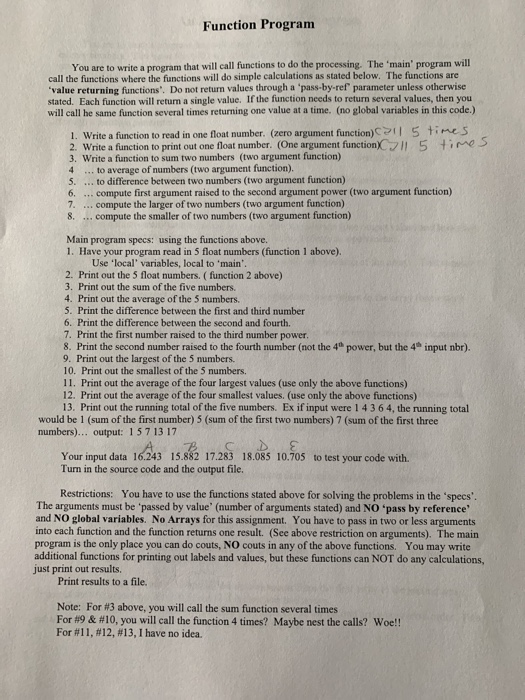Function Program You are to write a program that will call functions to do the processing. The &#39;main&#39; program will call the functions where the functions will do simple calculations as stated below. The functions are “value returning functions&#39;. Do not return values through a &#39;pass-by-ref parameter unless otherwise stated. Each function will return a single value. If the function needs to return several values, then you will call he same function several times returning one value at a time. (no global variables in this code.) 1. Write a function to read in one float number. (zero argument function) 1 5 times 2. Write a function to print out one float number. (One argument function ] 5 time 3. Write a function to sum two numbers (two argument function) 4 … to average of numbers (two argument function). 5. … to difference between two numbers (two argument function) 6. … compute first argument raised to the second argument power (two argument function) 7. … compute the larger of two numbers (two argument function) 8. … compute the smaller of two numbers (two argument function) Main program specs: using the functions above. 1. Have your program read in 5 float numbers (function 1 above). Use &#39;local variables, local to &#39;main&#39;. 2. Print out the 5 float numbers. (function 2 above) 3. Print out the sum of the five numbers. 4. Print out the average of the 5 numbers. 5. Print the difference between the first and third number 6. Print the difference between the second and fourth. 7. Print the first number raised to the third number power. 8. Print the second number raised to the fourth number (not the 4 power, but the input nbr). 9. Print out the largest of the 5 numbers. 10. Print out the smallest of the 5 numbers. 11. Print out the average of the four largest values (use only the above functions) 12. Print out the average of the four smallest values. (use only the above functions) 13. Print out the running total of the five numbers. Ex if input were 1 4 3 6 4, the running total would be 1 (sum of the first number) 5 (sum of the first two numbers) 7 (sum of the first three numbers)… output: 157 13 17 ” Your input data 16.243 15.882 17.283 18.085 10.705 to test your code with Turn in the source code and the output file. Restrictions: You have to use the functions stated above for solving the problems in the &#39;specs&#39; The arguments must be passed by value&#39; (number of arguments stated) and NO &#39;pass by reference and NO global variables. No Arrays for this assignment. You have to pass in two or less arguments into each function and the function returns one result. (See above restriction on arguments). The main program is the only place you can do couts, NO couts in any of the above functions. You may write additional functions for printing out labels and values, but these functions can NOT do any calculations, just print out results. Print results to a file. Note: For #3 above, you will call the sum function several times For #9 &#10, you will call the function 4 times? Maybe nest the calls? Woe!! For #11, #12 #13, I have no idea.